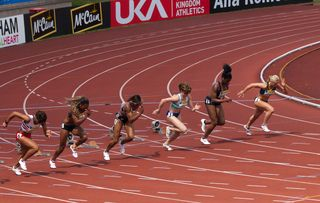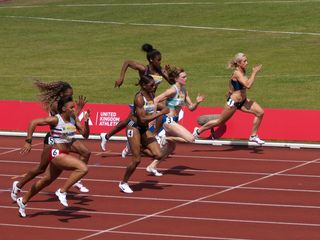We think you are located in United States. Is this correct?

# Test yourself now

High marks in science are the key to your success and future plans. Test yourself and learn more on Siyavula Practice.

## 21.5 Instantaneous velocity and speed (ESAGZ)

Sprinters taking offSprinters finishing raceWe have looked at the average velocity and speed but sometimes we want to be more precise about what is happening between the initial and final times in a problem.

Instantaneous velocity is the velocity at a specific instant in time. This can be different to the average velocity if the velocity isn't constant.

Look at the photographs of the sprinters in a race. Their velocity is different as they take off and as they end. Their average velocity for the race doesn't change but their instantaneous velocity, as captured in the “snapshots” of an instant in time does change. Their velocity in the photograph would be an instantaneous velocity.

An instant in time is different from the time taken or the time interval. It is therefore useful to use the symbol $$t$$ for an instant in time (for example during the fourth second) and the symbol $$\Delta t$$ for the time taken (for example during the first $$\text{5}$$ seconds of the motion).

Instantaneous velocity

Instantaneous velocity is the change in position over the change in a very small time interval ($$\Delta t\approx 0$$)

Quantity: Instantaneous velocity ($$\vec{v}$$) Unit name: metre per second Unit symbol: $$\text{m·s^{-1}}$$

Instantaneous speed

Instantaneous speed is the magnitude of instantaneous velocity.

Quantity: Instantaneous speed ($$v$$)         Unit name: metre per second         Unit symbol: $$\text{m·s^{-1}}$$

Instantaneous velocity is a vector. Instantaneous speed is the magnitude of instantaneous velocity. It has the same value but is not a vector so it has no direction.